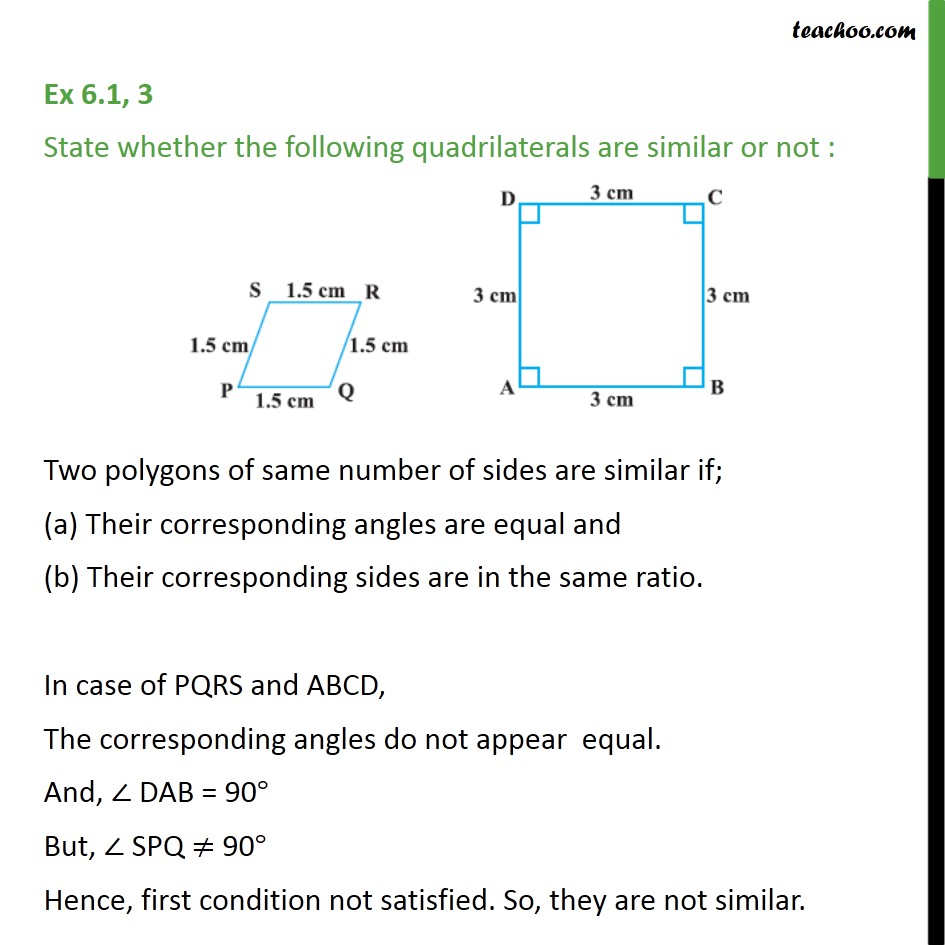1. Chapter 6 Class 10 Triangles
2. Serial order wise
3. Ex 6.1

Transcript

Ex 6.1, 3 State whether the following quadrilaterals are similar or not : Two polygons of same number of sides are similar if; (a) Their corresponding angles are equal and (b) Their corresponding sides are in the same ratio. In case of PQRS and ABCD, The corresponding angles do not appear equal. And, ∠ DAB = 90° But, ∠ SPQ ≠ 90° Hence, first condition not satisfied. So, they are not similar.

Ex 6.1

Chapter 6 Class 10 Triangles
Serial order wise